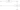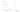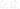Reading Notes - Ch. 4 - Fastbook (part 2)

Recall: Arthur Samuel's description of machine learning

Suppose we arrange for some automatic means of testing the effectiveness of any current weight assignment in terms of actual performance and provide a mechanism for altering the weight assignment so as to maximize the performance. We need not go into the details of such a procedure to see that it could be made entirely automatic and to see that a machine so programmed would “learn” from its experience.

Gradient descent is the "mechanism" that we use to alter the weight assignment (i.e. parameters of our model) so that we can maximize its performance (i.e. minimize the loss value).

The following diagram shows the different components involved in training a deep learning model:In this setup, we can use calculus to calculate how we should update the parameters of the model so that the loss value decreases. This change we have to make in the parameters of the model is given by the gradient of the loss function with respect to the parameters.

Technically, the gradient specifies the direction of steepest increase of the loss function. So we update the parameters in the negative direction of the gradient - i.e. the steepest decrease.After finding the gradient, we adjust the parameters of the model in the direction specified by the gradient to reduce the loss value, hence the name gradient descent.We have a way of improving the weights using gradient descent so it's completely fine to start with randomly initialized weights.

We stop training the model when we see that the accuracy of the model starts getting worse or we run out of time.

Batch, Mini-batch and Stochastic Gradient Descent

When calculating the gradient of the loss function with respect to the model parameters, at each step you can use all the data in the training set (batch gradient descent), one data point from the training set (stochastic gradient descent), or a subset of data (i.e. a mini-batch) in the training set (mini-batch gradient descent).

Batch gradient descent gives the most accurate gradient. However, computing the gradient over the entire dataset takes a long time. Also, it may not be possible to fit all your data in memory (especially a GPU) to use batch gradient descent if you have a very large dataset.

Stochastic gradient descent is the fastest since the gradient has to be calculated using only one point. However, it gives a very imprecise and unstable gradient. Also, using one data point at a time doesn't allow you to make full use of the parallel processing capabilities of a GPU.

Mini-batch gradient descent is a compromise between batch and stochastic gradient descent. A larger batch size (number of items in a mini-batch) gives a more accurate and stable estimate of the gradient, however smaller batches are faster to compute and allows you to update the gradient more number of times in an epoch.

Note: randomly shuffling items in mini-batches after every epoch can result in better generalization.

Learning Rate

The learning rate determines how big of a step we take when updating the weights. If it is too small, you will have to train the model for longer to reach the minima of the loss function. However, if it is too high then the loss will keep overshooting the minima. In fact, if the learning rate is sufficiently high, the loss can even start increasing.

The learning rate is used to control the weight update as follows:

New parameters = Old parameters - (gradient x learning rate)

The Loss Function

The choice of loss function is important for the gradient descent process to work. The loss function needs to update even when we make slight adjustments to our model parameters.

This is why accuracy is not a good choice for a loss function. If we change our weights a little bit, the output of the model may not change significantly and therefore its accuracy may not change. This will result in a gradient value of zero and our model won't be able to learn at that step.

Nonlinearities

A linear classifier is one whose output is a function of the linear combination of the model's parameters and input features. Adding multiple linear layers one after another can't improve the performance of a model because multiple linear layers, one after another, are equivalent to a single linear layer (with different parameters).

Adding a nonlinearity (also called an "activation function") between linear layers decouples the layers and enables each layer to learn on its own.

Two examples of nonlinearities are the ReLU and Sigmoid functionsThe Universal Approximation Theorem

For any arbitrarily wiggly function, we can approximate it as a bunch of lines joined together; to make it close to the wiggly function, we just have to use shorter lines.

Deeper Models

We can build deep models using multiple linear layers with a nonlinearity between them. However, the deeper a model gets, the harder it is to optimize its parameters in practice.

The advantage of using deep models is that you can use a deeper model with fewer parameters to get the same performance as a shallower model with more parameters.

As a result, deeper models can be trained faster and using less memory.